Next: Bibliography Up: Dissertation Otmar Ertl Previous: B.2 Root Finding

# C. Inequalities

Inequality 1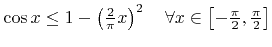(C.1)

Proof. According to the Weierstraß factorization theorem, the cosine function can be written as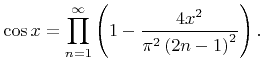(C.2)

Bearing in mind that all factors are in the range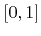for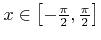, the inequality follows directly by neglecting all factors with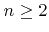. The first factor is identical to the right-hand side in (C.1).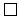Inequality 2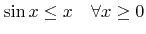(C.3)

Proof. For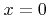both sides are equal. Hence, it is sufficient to show that the function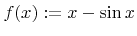is monotonically increasing. This is the case, if the first derivative is always non-negative,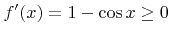, which is obviously satisfied.Inequality 3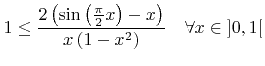(C.4)

Proof. The denominator is positive for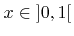. Thus, the inequality is equivalent to the next statement which is proved in the following for all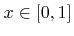: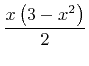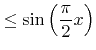(C.5)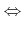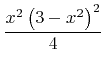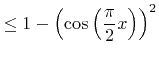(C.6)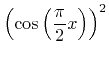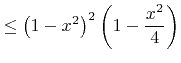(C.7)

Using the product representation of the cosine function (C.2):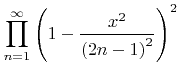(C.8)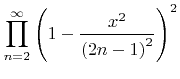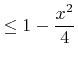(C.9)

Since all factors of this product series are in the range, it is sufficient to show that: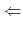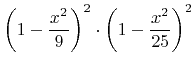(C.10)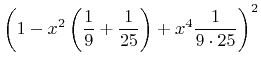(C.11)

Using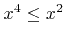for all: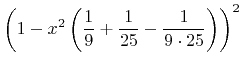(C.12)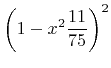(C.13)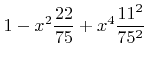(C.14)

Using again: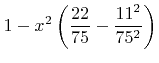(C.15)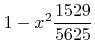(C.16)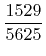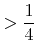(C.17)Next: Bibliography Up: Dissertation Otmar Ertl Previous: B.2 Root Finding

Otmar Ertl: Numerical Methods for Topography Simulation